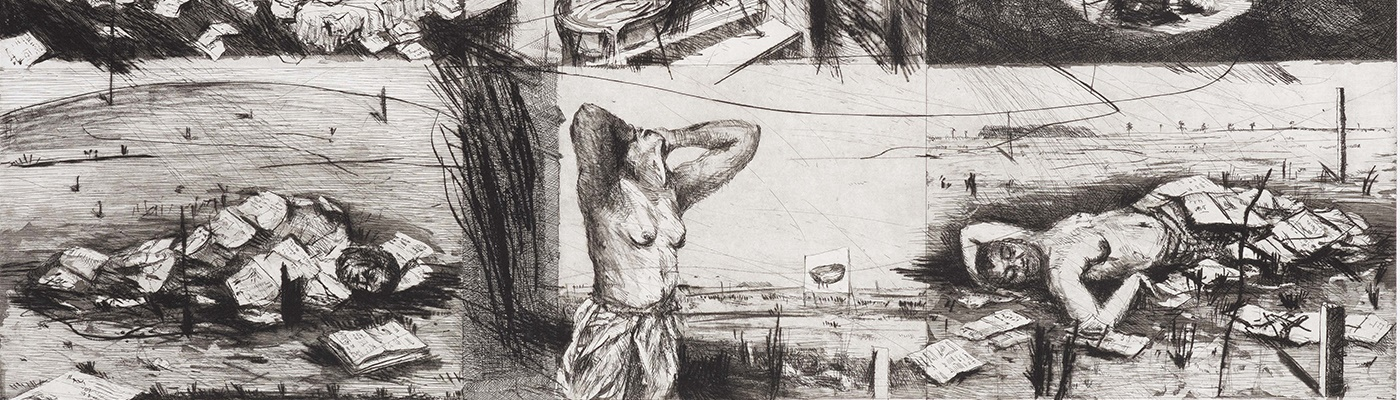##### Aspire X The Caversham Press 2020
Date: 27th Mar, 2020 11:00
Location: Online
Catalogue: View e-Catalogue

Name: Mrs Jacqui Carney
Email: jacqui@aspireart.net
Telephone: +27 71 675 2991

Category

Sold for R46,900

Estimated at R40,000 - R60,000

Estimated at R30,000 - R50,000

Sold for R158,288

Estimated at R150,000 - R200,000

Estimated at R35,000 - R45,000

Sold for R30,485

Estimated at R20,000 - R30,000

Estimated at R10,000 - R20,000

Sold for R234,500

Estimated at R200,000 - R300,000

Sold for R58,625

Estimated at R45,000 - R60,000

Sold for R6,449

Estimated at R5,000 - R10,000

Sold for R21,105

Estimated at R5,000 - R10,000

Sold for R4,690

Estimated at R4,000 - R8,000

Sold for R3,518

Estimated at R3,000 - R5,000

Sold for R4,104

Estimated at R3,000 - R5,000

Estimated at R15,000 - R25,000

Estimated at R6,000 - R10,000

Estimated at R6,000 - R10,000

Sold for R21,105

Estimated at R20,000 - R30,000

Sold for R28,140

Estimated at R25,000 - R35,000

Sold for R46,900

Estimated at R20,000 - R30,000

Sold for R23,450

Estimated at R15,000 - R20,000

Sold for R16,415

Estimated at R15,000 - R20,000

Sold for R44,555

Estimated at R35,000 - R45,000

Sold for R644,875

Estimated at R350,000 - R450,000

Estimated at R10,000 - R20,000

Estimated at R3,000 - R6,000

Sold for R6,449

Estimated at R5,000 - R10,000

Estimated at R15,000 - R25,000

Estimated at R4,000 - R8,000

Estimated at R6,000 - R10,000

Estimated at R55,000 - R65,000

Sold for R3,518

Estimated at R3,000 - R6,000

Sold for R5,862

Estimated at R5,000 - R10,000

Sold for R44,555

Estimated at R35,000 - R45,000

Estimated at R25,000 - R35,000

Estimated at R35,000 - R45,000

Estimated at R8,000 - R15,000

Estimated at R4,000 - R8,000

Estimated at R4,000 - R8,000

Estimated at R4,000 - R8,000

Sold for R117,250

Estimated at R100,000 - R150,000

Sold for R5,276

Estimated at R2,000 - R4,000

Estimated at R4,000 - R8,000

Estimated at R3,000 - R6,000

Sold for R93,800

Estimated at R80,000 - R120,000

Estimated at R5,000 - R10,000

Estimated at R800 - R1,200

Estimated at R4,000 - R8,000

Estimated at R6,000 - R10,000